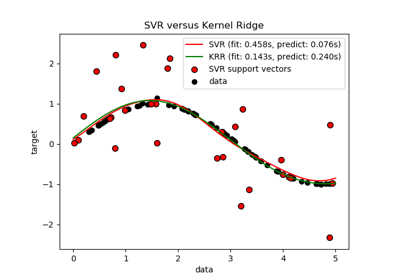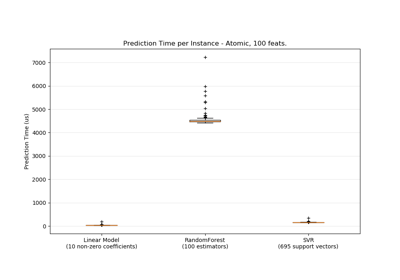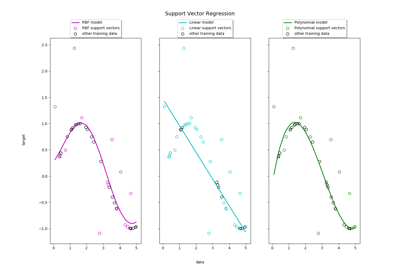# sklearn.svm.SVR¶

class sklearn.svm.SVR(kernel='rbf', degree=3, gamma='scale', coef0=0.0, tol=0.001, C=1.0, epsilon=0.1, shrinking=True, cache_size=200, verbose=False, max_iter=-1)[source]

Epsilon-Support Vector Regression.

The free parameters in the model are C and epsilon.

The implementation is based on libsvm. The fit time complexity is more than quadratic with the number of samples which makes it hard to scale to datasets with more than a couple of 10000 samples. For large datasets consider using sklearn.svm.LinearSVR or sklearn.linear_model.SGDRegressor instead, possibly after a sklearn.kernel_approximation.Nystroem transformer.

Read more in the User Guide.

Parameters
kernelstring, optional (default=’rbf’)

Specifies the kernel type to be used in the algorithm. It must be one of ‘linear’, ‘poly’, ‘rbf’, ‘sigmoid’, ‘precomputed’ or a callable. If none is given, ‘rbf’ will be used. If a callable is given it is used to precompute the kernel matrix.

degreeint, optional (default=3)

Degree of the polynomial kernel function (‘poly’). Ignored by all other kernels.

gamma{‘scale’, ‘auto’} or float, optional (default=’scale’)

Kernel coefficient for ‘rbf’, ‘poly’ and ‘sigmoid’.

• if gamma='scale' (default) is passed then it uses 1 / (n_features * X.var()) as value of gamma,

• if ‘auto’, uses 1 / n_features.

Changed in version 0.22: The default value of gamma changed from ‘auto’ to ‘scale’.

coef0float, optional (default=0.0)

Independent term in kernel function. It is only significant in ‘poly’ and ‘sigmoid’.

tolfloat, optional (default=1e-3)

Tolerance for stopping criterion.

Cfloat, optional (default=1.0)

Regularization parameter. The strength of the regularization is inversely proportional to C. Must be strictly positive. The penalty is a squared l2 penalty.

epsilonfloat, optional (default=0.1)

Epsilon in the epsilon-SVR model. It specifies the epsilon-tube within which no penalty is associated in the training loss function with points predicted within a distance epsilon from the actual value.

shrinkingboolean, optional (default=True)

Whether to use the shrinking heuristic.

cache_sizefloat, optional

Specify the size of the kernel cache (in MB).

verbosebool, default: False

Enable verbose output. Note that this setting takes advantage of a per-process runtime setting in libsvm that, if enabled, may not work properly in a multithreaded context.

max_iterint, optional (default=-1)

Hard limit on iterations within solver, or -1 for no limit.

Attributes
support_array-like of shape (n_SV)

Indices of support vectors.

support_vectors_array-like of shape (n_SV, n_features)

Support vectors.

dual_coef_array, shape = [1, n_SV]

Coefficients of the support vector in the decision function.

coef_array, shape = [1, n_features]

Weights assigned to the features (coefficients in the primal problem). This is only available in the case of a linear kernel.

coef_ is readonly property derived from dual_coef_ and support_vectors_.

fit_status_int

0 if correctly fitted, 1 otherwise (will raise warning)

intercept_array, shape = 

Constants in decision function.

NuSVR

Support Vector Machine for regression implemented using libsvm using a parameter to control the number of support vectors.

LinearSVR

Scalable Linear Support Vector Machine for regression implemented using liblinear.

Notes

Examples

>>> from sklearn.svm import SVR
>>> import numpy as np
>>> n_samples, n_features = 10, 5
>>> rng = np.random.RandomState(0)
>>> y = rng.randn(n_samples)
>>> X = rng.randn(n_samples, n_features)
>>> clf = SVR(C=1.0, epsilon=0.2)
>>> clf.fit(X, y)
SVR(epsilon=0.2)


Methods

 fit(self, X, y[, sample_weight]) Fit the SVM model according to the given training data. get_params(self[, deep]) Get parameters for this estimator. predict(self, X) Perform regression on samples in X. score(self, X, y[, sample_weight]) Return the coefficient of determination R^2 of the prediction. set_params(self, \*\*params) Set the parameters of this estimator.
__init__(self, kernel='rbf', degree=3, gamma='scale', coef0=0.0, tol=0.001, C=1.0, epsilon=0.1, shrinking=True, cache_size=200, verbose=False, max_iter=-1)[source]

Initialize self. See help(type(self)) for accurate signature.

fit(self, X, y, sample_weight=None)[source]

Fit the SVM model according to the given training data.

Parameters
X{array-like, sparse matrix}, shape (n_samples, n_features)

Training vectors, where n_samples is the number of samples and n_features is the number of features. For kernel=”precomputed”, the expected shape of X is (n_samples, n_samples).

yarray-like, shape (n_samples,)

Target values (class labels in classification, real numbers in regression)

sample_weightarray-like, shape (n_samples,)

Per-sample weights. Rescale C per sample. Higher weights force the classifier to put more emphasis on these points.

Returns
selfobject

Notes

If X and y are not C-ordered and contiguous arrays of np.float64 and X is not a scipy.sparse.csr_matrix, X and/or y may be copied.

If X is a dense array, then the other methods will not support sparse matrices as input.

get_params(self, deep=True)[source]

Get parameters for this estimator.

Parameters
deepbool, default=True

If True, will return the parameters for this estimator and contained subobjects that are estimators.

Returns
paramsmapping of string to any

Parameter names mapped to their values.

predict(self, X)[source]

Perform regression on samples in X.

For an one-class model, +1 (inlier) or -1 (outlier) is returned.

Parameters
X{array-like, sparse matrix}, shape (n_samples, n_features)

For kernel=”precomputed”, the expected shape of X is (n_samples_test, n_samples_train).

Returns
y_predarray, shape (n_samples,)
score(self, X, y, sample_weight=None)[source]

Return the coefficient of determination R^2 of the prediction.

The coefficient R^2 is defined as (1 - u/v), where u is the residual sum of squares ((y_true - y_pred) ** 2).sum() and v is the total sum of squares ((y_true - y_true.mean()) ** 2).sum(). The best possible score is 1.0 and it can be negative (because the model can be arbitrarily worse). A constant model that always predicts the expected value of y, disregarding the input features, would get a R^2 score of 0.0.

Parameters
Xarray-like of shape (n_samples, n_features)

Test samples. For some estimators this may be a precomputed kernel matrix or a list of generic objects instead, shape = (n_samples, n_samples_fitted), where n_samples_fitted is the number of samples used in the fitting for the estimator.

yarray-like of shape (n_samples,) or (n_samples, n_outputs)

True values for X.

sample_weightarray-like of shape (n_samples,), default=None

Sample weights.

Returns
scorefloat

R^2 of self.predict(X) wrt. y.

Notes

The R2 score used when calling score on a regressor will use multioutput='uniform_average' from version 0.23 to keep consistent with r2_score. This will influence the score method of all the multioutput regressors (except for MultiOutputRegressor). To specify the default value manually and avoid the warning, please either call r2_score directly or make a custom scorer with make_scorer (the built-in scorer 'r2' uses multioutput='uniform_average').

set_params(self, **params)[source]

Set the parameters of this estimator.

The method works on simple estimators as well as on nested objects (such as pipelines). The latter have parameters of the form <component>__<parameter> so that it’s possible to update each component of a nested object.

Parameters
**paramsdict

Estimator parameters.

Returns
selfobject

Estimator instance.

## Examples using sklearn.svm.SVR¶